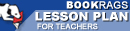Lesson Plans

# Chaos: Making a New Science Quiz | Eight Week Quiz G

This set of Lesson Plans consists of approximately 131 pages of tests, essay questions, lessons, and other teaching materials.
 View a FREE sampleName: _________________________ Period: ___________________

This quiz consists of 5 multiple choice and 5 short answer questions through chapters 9-11.

## Multiple Choice Questions

1. From what institution did Thomas S. Kuhn earn his B.S., M.S. and Ph.D. degrees in physics?
(a) Princeton University.
(b) Harvard University.
(c) Loyola University.
(d) Stanford University.

2. In fluid dynamics, what condition for viscous fluids states that at a solid boundary, the fluid will have zero velocity relative to the boundary?
(a) Chaos theory.
(b) Momentum diffusion.
(c) Period doubling bifurcation.
(d) No-slip condition.

3. What can be formed by taking a finite Cartesian product of the Cantor set with itself, making it a Cantor space?
(a) Cantor dust.
(b) Euclidean space.
(c) Helium in a Box.
(d) Fractal.

4. James Yorke realized that one of the largest problems with his work was that mathematicians and physicists were often worlds apart because they did not what?
(a) Use the same equipment.
(b) Speak the same language.
(d) Report to work at the same time.

5. Where was Mitchell Feigenbaum born?
(a) Hartford, Connecticut.
(b) New York, New York.
(c) Biloxi, Mississippi.
(d) Frankfort, Kentucky.

1. What is a measure of the resistance of a fluid which is being deformed by either shear stress or tensile stress?

2. What is a sub-discipline of fluid mechanics that deals with the natural science of fluids (liquids and gases) in motion?

3. What is a concept in mathematics where a fixed rule describes the time dependence of a point in a geometrical space?

4. What book did Michael Barnsley publish in 1988?

5. Who is attributed with the following quote in Chapter 4, "A Geometry of Nature": "And yet relation appears, a small relation expanding like the shade of a cloud on sand, a shape on the side of the hill"?

 This section contains 298 words (approx. 1 page at 300 words per page) View a FREE sample